# Non Verbal Reasoning - Rule Detection

Exercise : Rule Detection - Section 2
Directions to Solve

In each of the following questions, choose the set of figures which follows the given rule.

1.

Choose the set of figures which follows the given rule.

Rule: Closed figures become more and more open and open figures become more and more closed.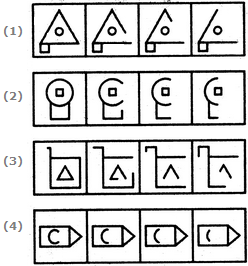1
2
3
4
Explanation:
No answer description is available. Let's discuss.

2.

Choose the set of figures which follows the given rule.

Rule: The series becomes simpler as it proceeds.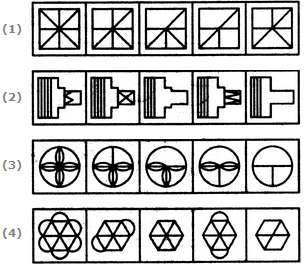1
2
3
4
Explanation:
No answer description is available. Let's discuss.

3.

Choose the set of figures which follows the given rule.

Rule: As the circle decreases in size, its sectors increase in number.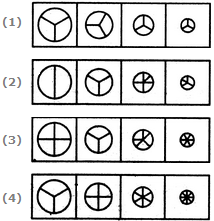1
2
3
4
Explanation:
No answer description is available. Let's discuss.

4.

Choose the set of figures which follows the given rule.

Rule: The series becomes complex as it proceeds.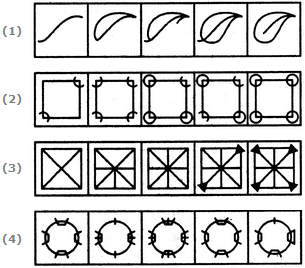1
2
3
4
Explanation:
No answer description is available. Let's discuss.

5.

Choose the set of figures which follows the given rule.

Rule: Closed figures become more and more open and open figures become more and more closed.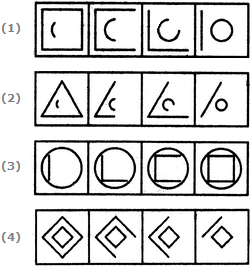1
2
3
4